# NCERT Solution for class 8 maths chapter 12 Exponents and Powers

NCERT solutions for class 8 maths chapter 12 Maths Exponents and Powers is prepared by academic team of Physics Wallah. we have prepared solutions for all exercise of chapter 12. Given below is step by step solutions of all questions given in NCERT textbook for chapter 12. Read chapter 12 theory make sure you have gone through the theory part of chapter 12 from NCERT textbook and you have learned the formula of the given chapter.Physics Wallah prepared a detail notes and additional questions for class 8 maths with short notes of all maths formula of class 8 maths. do read these contents before moving to solve the exercise of NCERT chapter 12. The NCERT solutions contains questions, answers, pictures and explanations for the complete Chapter 12 Exponents and Powers From of Science taught in Class 8.

### NCERT Solutions for Class 8 Maths Exercise 12.1

Question 1.

Evaluate:

(i)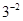(ii)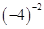(iii)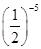Solution :

(i)= 1/3²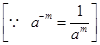= 1/9

(ii)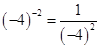= 1/16

(iii)=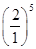=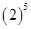= 32

Question 2.

Simplify and express the result in power notation with positive exponent:

(i)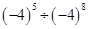(ii)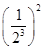(iii)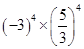(iv)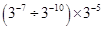(v)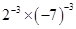Solution :

(i)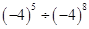=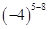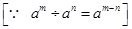=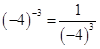(ii)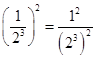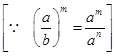=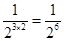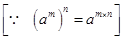(iii)=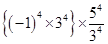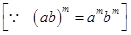=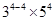=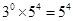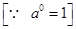(iv)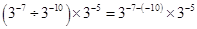=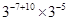=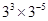=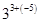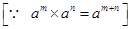=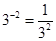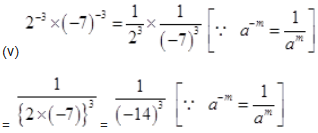Question 3.

Find the value of: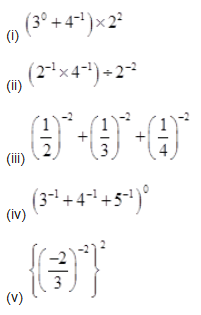Solution :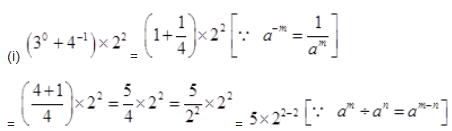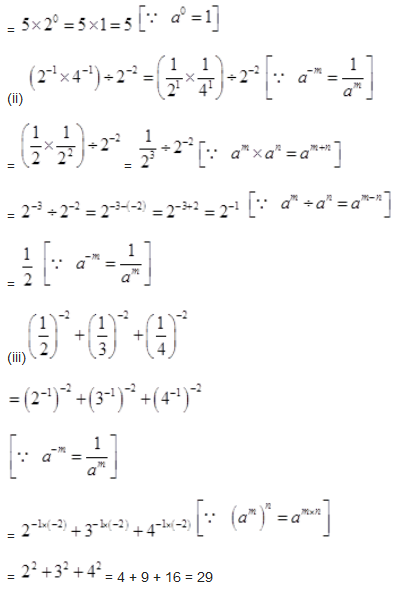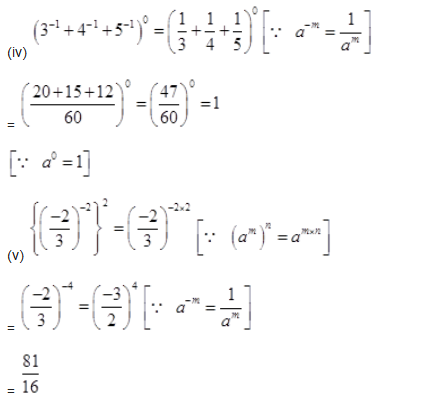Question 4.

Evaluate: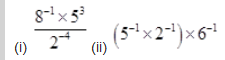Solution :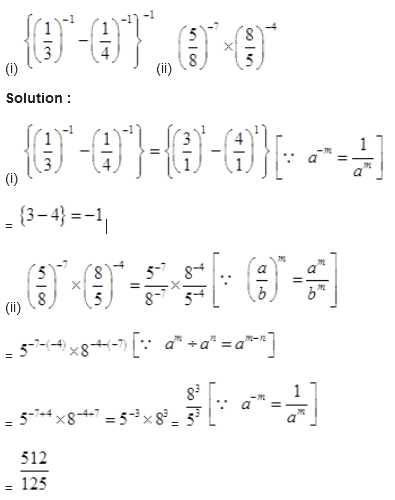= 1/10 × 1/6 = 1/60

Question 5.
Find the value of m for which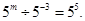Solution :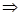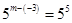Comparing exponents both sides, we getm+3 = 5m = 5 - 3m = 2

Question 6.
Evaluate: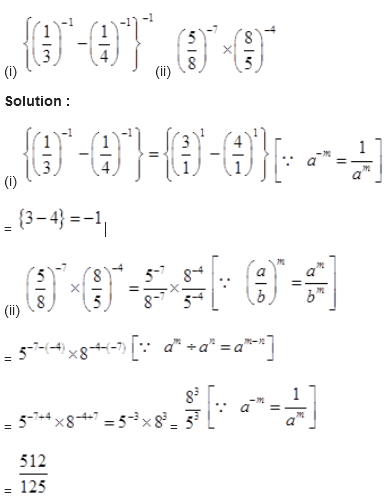Question 7.
Simplify: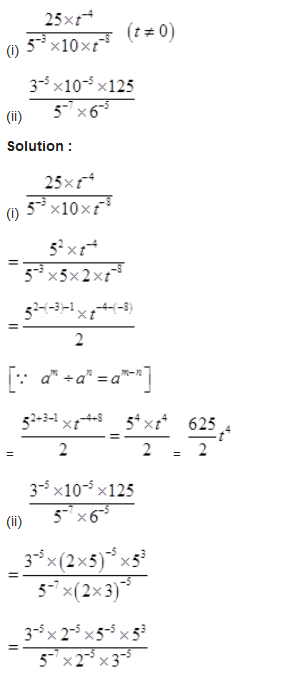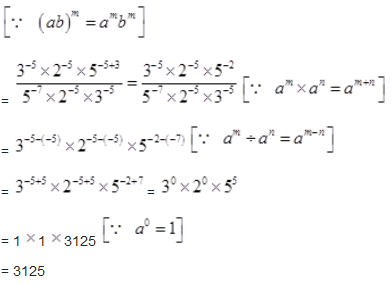### NCERT Solutions for Class 8 Maths Exercise 12.2

Question 1.

Express the following numbers in standard form:

(i) 0.0000000000085

(ii) 0.00000000000942

(iii) 6020000000000000

(iv) 0.00000000837

(v) 31860000000

Solution :

(i) 0.0000000000085

= 0.0000000000085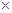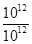=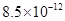(ii) 0.00000000000942

= 0.00000000000942=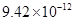(iii) 6020000000000000

= 6020000000000000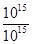=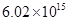(iv) 0.00000000837

= 0.00000000837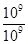=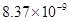(v) 31860000000 = 31860000000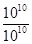=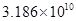Question 2.

Express the following numbers in usual form :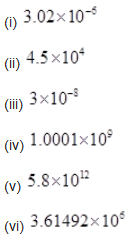Solution :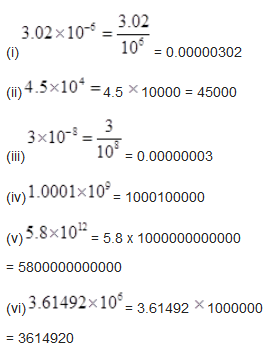Question 3.

Express the number appearing in the following statements in standard form :

(i)1 micron is equal to 1/ 1000000 m.

(ii) Charge of an electron is 0.000,000,000,000,000,000,16 coulomb.

(iii) Size of a bacteria is 0.0000005 m.

(iv) Size of a plant cell is

0.00001275 m.

(v) Thickness if a thick paper is 0.07 mm.

Solution :

(i) 1 micron

=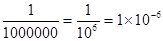m

(ii)Charge of an electron is

0.00000000000000000016 coulombs.

= 0.00000000000000000016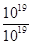=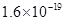coulomb

(iii) Size of bacteria = 0.0000005

=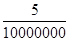=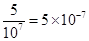m

(iv) Size of a plant cell is 0.00001275 m

= 0.00001275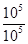=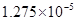m

(v) Thickness of a thick paper = 0.07 mm

= 7/100mm =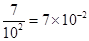mm

Question 4.

In a stack there are 5 books each of thickness 20 mm and 5 paper sheets each of thickness 0.016 mm. What is the total thickness of the stack?

Solution :

Thickness of one book = 20 mm

Thickness of 5 books = 20 × 5 = 100 mm

Thickness of one paper = 0.016 mm

Thickness of 5 papers = 0.016 × 5

= 0.08 mm

Total thickness of a stack = 100 + 0.08

=100.08 mm

= 100.08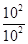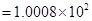mm

### Notes,worksheet and solved question for Maths class 8

Check your marks in a chapter which you have complited in school from Physics Wallah chapter wise online test just click on the link given below